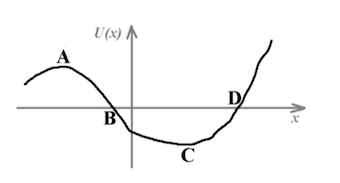# Problem: The plot shown represents the potential energy of a particle that can only move along the x-axis, as a function of the coordinate x. Which of the following statements is correct:[a] In B and D the force is zero;[b] The force in B is positive;[c] A and C are stable equilibrium positions;[d] The force in D is positive;[e] A and C are unstable equilibrium positions.

###### FREE Expert Solution
91% (151 ratings)
###### Problem Details

The plot shown represents the potential energy of a particle that can only move along the x-axis, as a function of the coordinate x. Which of the following statements is correct:

[a] In B and D the force is zero;

[b] The force in B is positive;

[c] A and C are stable equilibrium positions;

[d] The force in D is positive;

[e] A and C are unstable equilibrium positions.